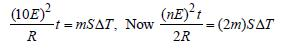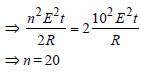Courses

# 28 Year NEET Questions: Current Electricity- 1

## 35 Questions MCQ Test Physics 28 Years Past year papers for NEET/AIPMT Class 12 | 28 Year NEET Questions: Current Electricity- 1

Description
This mock test of 28 Year NEET Questions: Current Electricity- 1 for NEET helps you for every NEET entrance exam. This contains 35 Multiple Choice Questions for NEET 28 Year NEET Questions: Current Electricity- 1 (mcq) to study with solutions a complete question bank. The solved questions answers in this 28 Year NEET Questions: Current Electricity- 1 quiz give you a good mix of easy questions and tough questions. NEET students definitely take this 28 Year NEET Questions: Current Electricity- 1 exercise for a better result in the exam. You can find other 28 Year NEET Questions: Current Electricity- 1 extra questions, long questions & short questions for NEET on EduRev as well by searching above.
QUESTION: 1

### In the circuit shown, if a conducting wire is connected between points A and B, the current in this wire will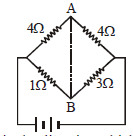Solution:

Current will flow from B to A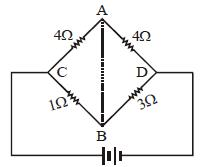Potential drop over the resistance CA will be
more due to higher value of resistance. So
potential at A will be less as compared with at
B. Hence, current will flow from B to A.

QUESTION: 2

### Kirchhoff’s first and second laws for electricalcircuits are consequences of 

Solution:

Kirchhoff ' s first law deals with conservation
of electrical charge & the second law deals
with conservation of electrical energy.

QUESTION: 3

### Two cells, having the same e.m.f., are connected in series through an external resistance R. Cells have internal resistances r1 and r2 (r1 > r2) respectively. When the circuit is closed, the potential difference across the first cell is zero. The value of R is [2006

Solution:

Current in the circuit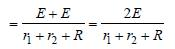P.D. across first cell = E – ir1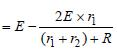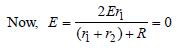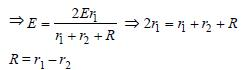QUESTION: 4

The total power dissipated in watts in the circuit shown here is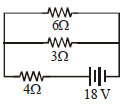Solution:

Power dissipiated = P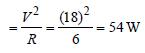QUESTION: 5

Three resistances P, Q, R each of 2 Ω  and an unknown resistance S form the four arms of a Wheatstone bridge circuit. When a resistance of 6 Ω is connected in parallel to S the bridge gets balanced. What is the value of S?

Solution: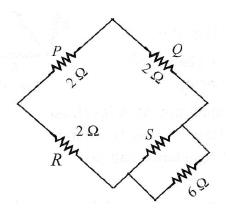For a balanced Wheatstone bridge, we get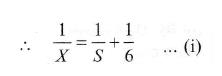Therefore, the equivalent circuit diagram drawn below.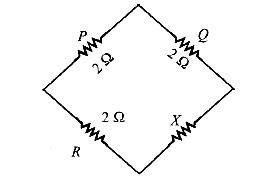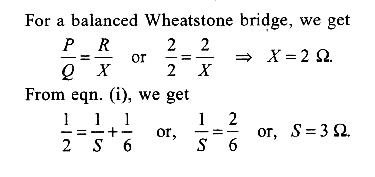QUESTION: 6

The resistance of an ammeter is 13 Ω and itsscale is graduated for a current upto 100 amps.After an additional shunt has been connectedto this ammeter it becomes possible to measurecurrents upto 750 amperes by this meter. Thevalue of shunt-resistance is 

Solution:

We know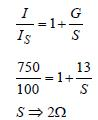QUESTION: 7

A steady current of 1.5 amp flows through acopper voltameter for 10 minutes. If the electrochemical equivalent of copper is30 × 10–5 g coulomb–1, the mass of copperdeposited on the electrode will be 

Solution:

We have, m = ZIt
where, Z is the electrochemical equivalent
of copper.
⇒ m = 30 x10-5 x1.5 x10 x 60
= 0.27 gm.

QUESTION: 8

A current of 3 amp flows through the 2Ω resistor shown in the circuit. The power dissipated in the 5-Ω resistor is: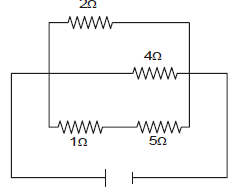Solution:

Clearly, 2Ω, 4Ω and ( 1 + 5) Ωresistors are
in parallel. Hence, potential difference is
same across each of them.
∴ I1 × 2 = I2 × 4 = I3 × 6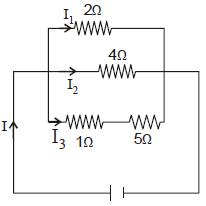Given I1 = 3A ∴ I1 × 2 = I3 × 6
Given I1 = 3A.
∴ I1 × 2 = I3 × 6 provides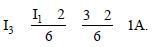Now, the potential across the 5Ω resistor is
V = I3 × 5 = 1 × 5 = 5V.
∴ the power dissipated in the 5Ωresistor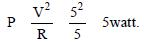QUESTION: 9

A wire of a certain material is stretched slowlyby ten per cent. Its new resistance and specificresistance become respectively: 

Solution:

Resistance of a wire is given by R  l/a

If the length is increased by 10% then new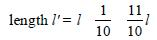In that case, area of cross-section of wire
would decrease by 10%
∴ New area of cross-section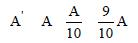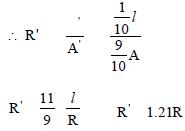Thus the new resistance increases by 1.21
times. The specific resistance (resistivity)
remains unchanged as it depends on the
nature of the material of the wire.

QUESTION: 10

A cell can be balanced against 110 cm and 100cm of potentiometer wire, respectively with andwithout being short circuited through aresistance of 10Ω. Its internal resistance is 

Solution:

Here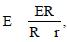hence the lengths 110 cm
and 100 cm are interchanged.
Without being short-circuited through R,
only the battery E is balanced.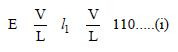When R is connected across E, Ri, V/L, l2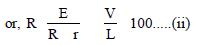Dividing (i) by (ii), we get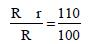or, 100 R + 100 r = 110 R
or, 10 R = 100 r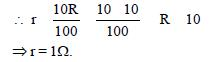QUESTION: 11

A galvanometer of resistance 50 Ω is connectedto battery of 3V along with a resistance of 2950 Ωin series. A full scale deflection of 30 divisions isobtained in the galvanometer. In order to reducethis deflection to 20 divisions, the resistance inseries should be 

Solution:

Total internal resistance = (50+2950)Ω
= 3000 Ω
Emf of the cell, ε = 3V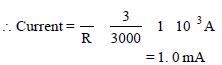Current for full scale deflection of 30
divisions is 1.0 mA.
∴ Current for a deflection of 20 divisions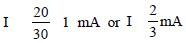Let the resistance be x Ω. Then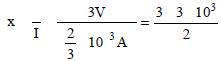= 4500 Ω
But the resistance of the galvanometer
is 50Ω
∴ Resistance to be added
= (4500 –50) Ω= 4450 Ω

QUESTION: 12

See the electric circuit shown in the figure.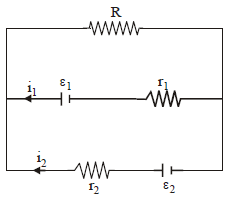Which of the following equations is a correct equation for it? 

Solution: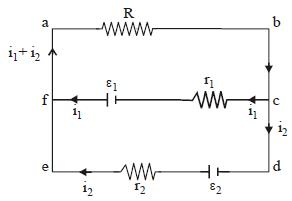Applying Kirchhoff ’s rule in loop abcfa
ε1 – (i1 + i2) R – i1 r1 = 0.

QUESTION: 13

A wire of resistance 12 Ωm-1 is bent to form a complete circle of radius 10 cm. The resistance between its two diametrically opposite point, A and B as shown in the figure, is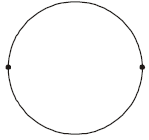Solution:

Total resistance of wire = 12Ω×2π×10−1
= 2.4π
Resistance of each half = 2.4π/2​=1.2π
and as about diameter both parts are in parallel
Req. = 1.2π/2​=0.6πΩ
The correct answer is option D.

QUESTION: 14

A student measures the terminal potentialdifference (V) of a cell (of emf E and internalresistance r) as a function of the current (I)flowing through it. The slope and intercept, ofthe graph between V and I, then, respectively,equal: 

Solution:

The terminal potential difference of a cell is
given by V + Ir = E

V= VA – VB
or V = E – Ir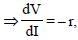Also for, i = 0 then V = E

∴ slope = – r, intercept = E

QUESTION: 15

A potentiometer circuit is set up as shown. The potential gradient, across the potentiometer wire, is k volt/cm and the ammeter, present in the circuit, reads 1.0 A when two way key is switched off. The balance points, when the key between the terminals (i) 1 and 2 (ii) 1 and 3, is plugged in, are found to be at lengths ℓ1 cm and ℓ2 cm respectively. The magnitudes, of the resistors R and X, in ohms, are then, equal, respectively, to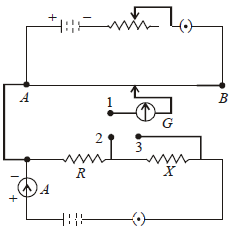Solution:

(i) When key between the terminals 1
and 2 is plugged in,
P.D. across R = IR = k l1
⇒ R = k l1 as I = 1A
(ii) When key between terminals 1 and 3 is
plugged in,
P.D. across (X + R) = I(X + R) = k l2
⇒ X + R = k l2
∴ X = k (l2 – l1)
∴ R = kl1 and X = k (l2 – l1)

QUESTION: 16

In producing chlorine by electrolysis 100 kWpower at 125 V is being consumed. How muchchlorine per minute is liberated? (E.C.E. ofchlorine is 0.367×10–6 kg / C) 

Solution:

Power = 100KW
Voltage = 125V
Power = Vi
100×103 = 125i
i = 100000/125
i = 800A
We know that first law of electrolysis
m = Zit (Z = E.C.E)
= 0.367/1000×10-6×800×60
= 367×48×10-6
= 17616×106 kg
= 17.6×10-3 kg

QUESTION: 17

Consider the following two statements:
(a) Kirchhoff's junction law follows from the conservation of charge.
(b) Kirchhoff's loop law follows from the conservation of energy.

Which of the following is correct? 

Solution:

Junction law follows from conservation
of charge and loop law is the conservation
of energy

QUESTION: 18

The thermo e.m.f E in volts of a certain thermocouple is found to vary with temperature difference θ in °C between the two junctions according to the relation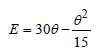The neutral temperature for the thermocouplewill be

Solution: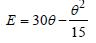For neutral temperature,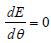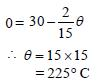Hence, neutral temperature is 225°C.

QUESTION: 19

A current of 2A flows through a 2Ω resistorwhen connected across a battery. The samebattery supplies a current of 0.5 A whenconnected across a 9Ω resistor. The internalresistance of the battery is 

Solution:

Let the internal resistance of the battery be
r. Then the current flowing through the
circuit is given by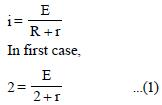⇒ 1.5 r  = 0.5 ⇒ r = 1/3Ω

QUESTION: 20

If power dissipated in the 9-Ω resistor in the circuit shown is 36 watt, the potential difference across the 2-Ω resistor is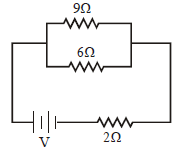Solution:

We have,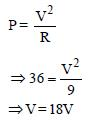Current passing through the 9Ω resistor is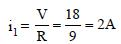The 9Ω and 6Ω resistors are in parallel,
therefore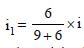where i is the current delivered by the
battery.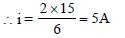Thus, potential difference across 2Ω
resistor is
V = iR
= 5 × 2
= 10V

QUESTION: 21

The rate of increase of thermo–e.m.f. withtemperature at the neutral temperature of athermocouple 

Solution:

We have,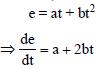At neutral temperature,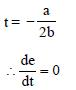QUESTION: 22

A thermocouple of negligible resistanceproduces an e.m.f. of 40 μV/°C in the linear rangeof temperature. A galvanometer of resistance 10ohm whose sensitivity is 1μA/div, is employedwith the termocouple. The smallest value oftemperature difference that can be detected bythe system will be [2011M]

Solution: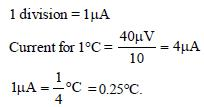QUESTION: 23

In the circuit shown in the figure, if the potential at point A is taken to be zero, the potential at point B is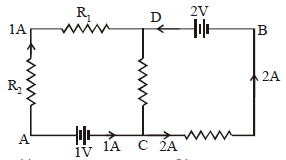Solution:

Current from D to C = 1A
∴ VD – VC = 2 × 1 = 2V
VA = 0     ∴ VC = 1V, ∴ VD – VC = 2
⇒VD – 1 = 2     ∴ VD = 3V
∴ VD – VB = 2 ∴ 3 – VB = 2 ∴ VB = 1V

QUESTION: 24

A milli voltmeter of 25 milli volt range is to beconverted into an ammeter of 25 ampere range.The value (in ohm) of necessary shunt will be :

Solution:

Galvanometer is converted into ammeter,
by connected a shunt, in parallel with it.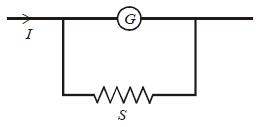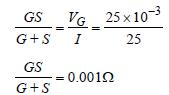Here S << G so
S = 0.001 Ω

QUESTION: 25

In the circuit shown the cells A and B have negligible resistances. For VA = 12V, R1 = 500Ω and R = 100Ω the galvanometer (G) shows no deflection. The value of VB is :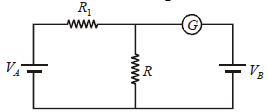Solution: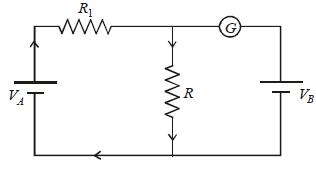Since deflection in galvanometer is zero so
current will flow as shown in the above
diagram.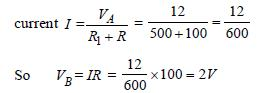QUESTION: 26

If voltage across a bulb rated 220 Volt-100 Wattdrops by 2.5% of its rated value, the percentageof the rated value by which the power woulddecrease is : 

Solution:

Resistance of bulb is constant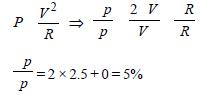QUESTION: 27

A ring is made of a wire having a resistance R0 = 12 Ω. Find the points A and B as shown in the figure, at which a current carrying conductor should be connected so that the resistance R of the sub-circuit between these points is equal to 8/3 Ω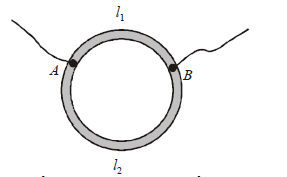Solution:

Let x is the resistance per unit length then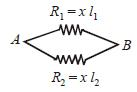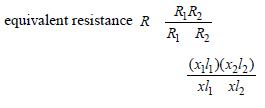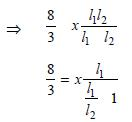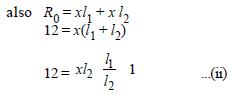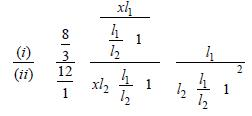(y2 + 1 + 2y) ×  8/36 y  (where  y l1/l2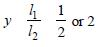QUESTION: 28

The power dissipated in the circuit shown in the figure is 30 Watts. The value of R is: [2012M]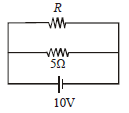Solution:

The power dissipated in the circuit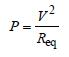v = 10 volt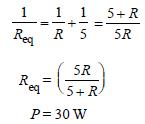Substituting the values in equation (i)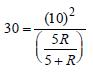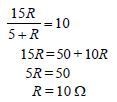QUESTION: 29

Cell having an emf ε and internal resistance r is connected across a variable external resistance R. As the resistance R is increased, the plot of potential difference V across R is given by : [2012M]

Solution:

The current through the resistance R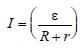The potential difference across R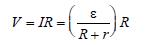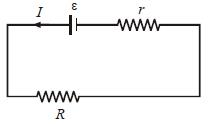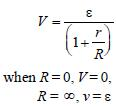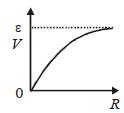Thus V increases as R increases upto certain
limit, but it does not increase further.

QUESTION: 30

A wire of resistance 4 Ω is stretched to twice itsoriginal length. The resistance of stretched wirewould be [NEET 2013]

Solution: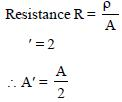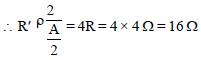Therefore the resistance of new wire
becomes 16 Ω

QUESTION: 31

The internal resistance of a 2.1 V cell which givesa current of 0.2 A through a resistance of 10 Ω is[NEET 2013]

Solution:

Given : emf ε = 2.1 V
I = 0.2 A, R = 10Ω
Internal resistance r = ?
From formula.
ε – Ir = V = IR
2.1 – 0.2r = 0.2 × 10
2.1 – 0.2 r = 2 or 0.2 r = 0.1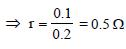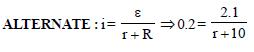⇒ 2.1 = 0.2r +2 ⇒ r = 1/2 = 0.5Ω

QUESTION: 32

The resistance of the four arms P, Q, R and S in a Wheatstone’s bridge are 10 ohm, 30 ohm, 30 ohm and 90 ohm, respectively. The e.m.f. and internal resistance of the cell are 7 volt and 5 ohmrespectively. If the galvanometer resistance is50 ohm, the current drawn from the cell will be [NEET 2013]

Solution:

Given : V = 7 V
r = 5Ω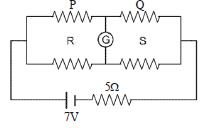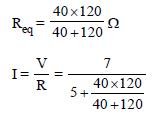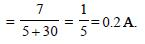QUESTION: 33

A 12 cm wire is given a shape of a right angled triangle ABC having sides 3 cm, 4 cm and 5 cm as shown in the figure. The resistance between two ends (AB, BC, CA) of the respective sides are measured one by one by a multi-meter. The resistances will be in the ratio of    [NEET Kar. 2013]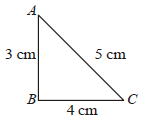Solution:

Resistance is directly proportional to length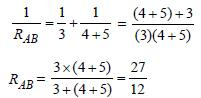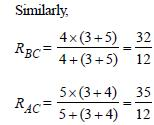∴ RAB : RBC : RAC = 27 : 32 : 35

QUESTION: 34

Two rods are joined end to end, as shown. Both have a cross-sectional area of 0.01 cm2. Each is 1 meter long. One rod is of copper with a resistivity of 1.7 × 10–6 ohm-centimeter, the other is of iron with a resistivity of 10–5 ohmcentimeter.     [NEET Kar. 2013]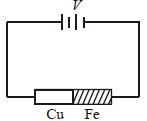How much voltage is required to produce a current of 1 ampere in the rods?

Solution:

Copper rod and iron rod are joined in series.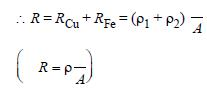From ohm’s law V = RI

= (1.7 × 10–6 × 10–2 + 10–5 × 10–2) ÷ 0.01 × 10–4 volt
= 0.117 volt ( I = 1A)

QUESTION: 35

Ten identical cells connected in series areneeded to heat a wire of length one meter andradius ‘r’ by 10ºC in time ‘t’. How many cells willbe required to heat the wire of length two meterof the same radius by the same temperature intime ‘t’? [NEET Kar. 2013]

Solution:

Resistance is directly proportionl to length
of the wire. As length is doubled so mass is
doubled and resistance is doubled.
We have